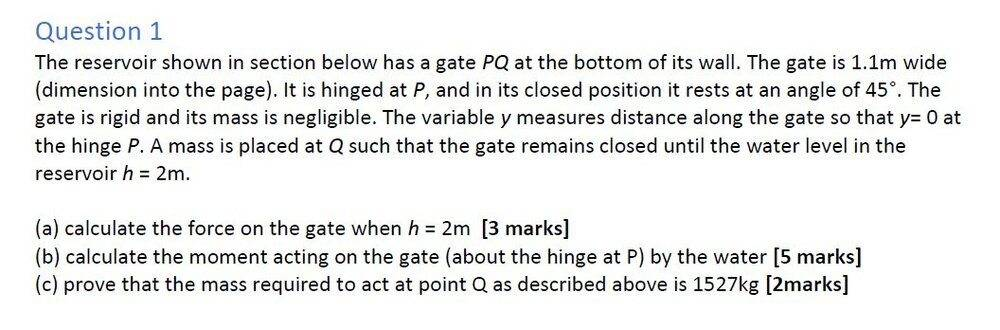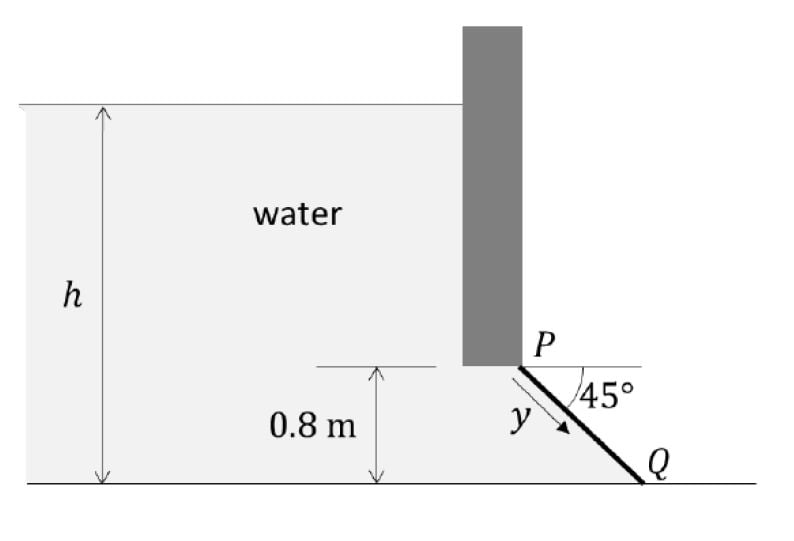# Hydrostatic Problem: Hinged water gate at the bottom of a reservoir

Homework Helper
Gold Member
Homework Statement:
See Below
Relevant Equations:My son and I are having a problem with part c that suggests our answer for part a and b are wrong..

Part a). If we've understood the teaching notes correctly the force on the gate acting through its centroid perpendicular to the gate and is given by

where
ρ is the density of water 997 kg/m^3
g is 9.81m/s^2
A is the area of the gate
d is the depth of the centroid of the gate

The area of the gate A is

A = 1.1 * 0.8/cos(45)
= 1.24 sqm

The centroid is half way up the gate so at a depth d below the surface of

d = 2-0.4
= 1.6m

So the Force F acting through the centroid is

F = 997 * 9.81 * 1.24 * 1.6
= 19,405 N

Part b) The centroid of the gate is at..

y = 0.5 * 0.8/cos(45)

so the moment M acting on the gate due to water pressure is

M = 19,405 * 0.5 * 0.8/cos(45)
= 10,977 Nm

Part c) If the gate isn't accelerating the net moment must be zero.

The clockwise moment due to the mass Q is

= 0.8*Q*g

The anti clockwise moment due to water pressure is 10,977 Nm from above.

Equating these gives

0.8*Q*g = 10,977

and

Q = 10,977/(0.8*g)
= 1,398 kg

So we have failed to prove mass Q needs to be 1,527 kg

Where are we going wrong?

Last edited by a moderator:

Homework Helper
misfired :)

Mentor
I think part b may not have been done right. If the pressure at y is p(y). the moment of the pressure force is $$M=\int{wyp(y)dy}$$This would not be equal to the force times the distance to the centroid.

•